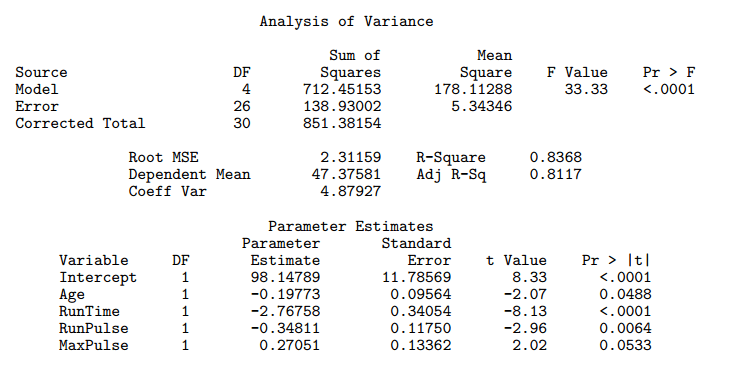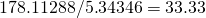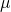## The meaning of F Value in the Analysis of Variance for Linear regression

This is a sample output for linear regression:The F Value is computed by dividing the value in the Mean Square column for Model with the value in the Mean Square column for Error. In our example, it’s.

There are two possible interpretations for the F Value in the Analysis of Variance table for the linear regression.

## On the meaning of hypothesis and p-value in statistical hypothesis testing

Statistical hypothesis testing is really an interesting topic. I’ll just briefly sum up what statistical hypothesis testing is about, and what you do to test an hypothesis, but will assume you are already familiar with it, so that I can quickly cover a couple of A-HAs moments I had.

In statistical hypothesis testing, we

• have some data, whatever it is, which we imagine as being values of some random variable;
• make an hypothesis about the data, such as that the expected value of the random variable is;
• find a distribution for any affine transformation of the random variable we are making inference about – this is the test statistic;
• run the test, i.e. numerically say how much probable how observations were in relation to the hypothesis we made.

I had a couple of A-HA moments I’d like to share.

There is a reason why this is called hypothesis testing and not hypothesis choice. There are indeed two hypothesis, the null and the alternative hypothesis. However, their roles are widely different! 90% of what we do, both from a conceptual and a numerical point of view, has to do with the null hypothesis. They really are not symmetric. The question we are asking is “With the data I have, am I certain enough my null hypothesis no longer stands?” not at all “With the data I have, which of the two hypothesis is better?”